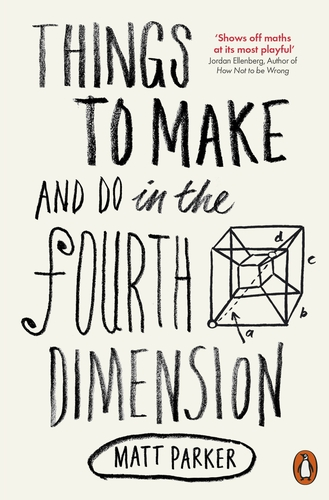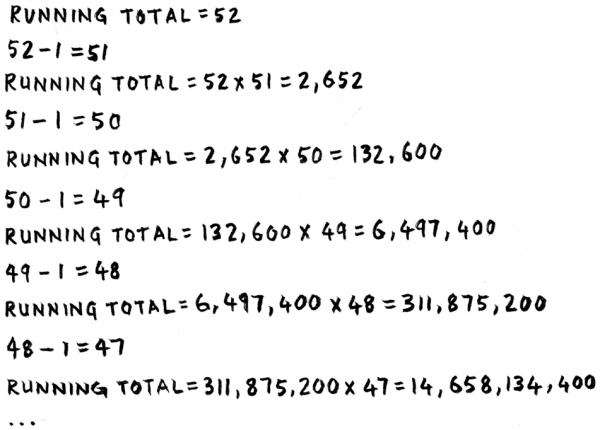# How many ways to shuffle a pack of cards?

This is an excerpt from friend of The Aperiodical, Matt Parker’s book, “Things to Make and Do in the Fourth Dimension”, which is out now in paperback.There’s a lovely function in mathematics called the factorial function, which involves multiplying the input number by every number smaller than it. For example: $\operatorname{factorial}(5) = 5 \times 4 \times 3 \times 2 \times 1 = 120$. The values of factorials get alarmingly big so, conveniently, the function is written in shorthand as an exclamation mark. So when a mathematician writes things like $5! = 120$ and $13! = 6,\!227,\!020,\!800$ the exclamation mark represents both factorial and pure excitement. Factorials are mathematically interesting for several reasons, possibly the most common being that they represent the ways objects can be shuffled. If you have thirteen cards to shuffle, then there are thirteen possible cards you could put down first. You then have the remaining twelve cards as options for the second one, eleven for the next, and so on – giving just over 6 billion possibilities for arranging a mere thirteen cards.

For a full deck of fifty-two cards, the number is much bigger. Calculating $52!$ manually would take a long time, so it’s a perfect thing to get a computer to do for us. But in order to ask a computer to do something, you need to state it as an algorithm for the computer to follow. So here’s a set of instructions I’ve written to take an input number and progressively multiply it by every smaller number:

Step 1: Remember the starting value of $n$ as your running total.

Step 2: Subtract $1$ from $n$.

Step 3: Multiply the running total by this new $n$.

Step 4: Repeat steps 2 and 3 until $n$ reaches $1$.

Step 5: Return the running total.

We can try running this for the first few laps of calculating $52!$:We’re only six laps in, and the running total has already gone past 14 billion! (No that’s not a factorial, I just wanted to emphasize how quickly these numbers grow.) This is definitely the sort of long calculation we want a computer to do for us. The last step is to translate our algorithm steps into a language a computer can understand. So what does an algorithm look like to a computer? Well, much as humans can speak different languages, computers can understand different programming languages. I’ve selected one called Python, because it has a very simple grammar and is one of the closest computer languages to being readable English. I’ll also include some comments to the right of each line to clarify
what the code is doing.

Here is the factorial algorithm, coded in Python. If you are so inclined, you could run this on a computer. Much in the way we were naming functions before, I can give each algorithm a name then write its inputs inside brackets next to it.

def factorial(n):       # I am defining the algorithm called
# ‘factorial’ and it starts with a number ‘n’
running_total = n    # Remembers n as the starting value of
# the running total
while n > 1:      # Repeats the next bit until n is no longer
# bigger than 1
n = n - 1            # Subtracts 1 from n
running_total =         # Multiplies the running total
running_total * n    # by the new n
return running_total    # Returns the running total


I’ve just double-checked this program to make sure it works. Once loaded up, I can enter factorial(13) into my computer to double-check $13!$. And, sure enough, $6,\!227,\!020,\!800$ appears
back on the screen. So then I let it loose on $52!$ and here is the exact output from my computer:

>>> factorial(52)
80658175170943878571660636856403766975289505440883277824000000000000


That’s a number with sixty-eight digits. A truly huge number. To say it the long way, there are 800,000 billion billion billion billion billion billion billion ways a pack of cards could be
shuffled. There are only about 1 million billion billion stars in the observable universe. And the universe is only about 4 hundred million billion seconds old. So this means that if every star in the universe had a billion planets, each with a population of a billion hypothetical aliens, all shuffling a billion packs a second since the dawn of the universe, we would now be only halfway through every possible arrangement. And that’s for a pack of only fifty-two cards! Thank goodness we didn’t include the jokers.

Anyway, now we know what the answer is, we can try to calculate it in a different way: using a recursive algorithm. We simply need to state the algorithm in terms of itself. Something like: ‘The factorial of $n$ is $n$ times the factorial of $n – 1$.’ The only extra thing we need to know is that the factorial of $1$ is $1$. This is the ‘get-out clause’ in the algorithm which stops the recursion going on for ever.

Step 1: Remember that the factorial of $1$ is $1$.

Step 2: Multiply $n$ by the factorial of $n – 1$.

Translated into Python:

def factorial(n):              # I am calling the algorithm ‘factorial’
# and it starts with a number ‘n’
if n == 1: return 1         # The factorial of 1 is 1
return n * factorial(n - 1) # Calculate n multiplied by the factorial
# of n - 1


Presented with factorial(13), my computer now runs down the chain of recursions until it hits factorial(1), then comes racing back up to give $6,\!227,\!020,\!800$ once more. Likewise, putting in factorial(52) gives back the same 68-digit monster. Yet nowhere have I actually told the program how to calculate a factorial, merely how one factorial calculation relates to a smaller one. Once again, recursive algorithms are magic: conjuring answers out of seemingly empty code.

We now have two different computer programs which compute the same answer two different ways. Which leaves us with only one option: make them fight! I just took a quick break to race both factorial programs head to head. I decided that the winner would be the first to compute the factorial of $100$. Computing the full 158-digit answer to $100!$ took the recursive Python program 0.000068 seconds, whereas the normal one came in at only 0.000046 seconds.1 A decisive win for the non-recursive program!

If you liked that, “Things to Make and Do in the Fourth Dimension” is out now in paperback and contains plenty more playful mathematical investigations. There’s also a fantastic website to accompany the book, with loads of interactive doodads, at makeanddo4d.com

1. 93326215443944152681699238856266700490715968264381621468592963895217599993229915608941463976156518286253697920827223758251185210916864000000000000000000000000, for the record. []

•#### Matt Parker

Maths. Stand-up. Whatnot.

### 5 Responses to “How many ways to shuffle a pack of cards?”

1.Iona

Woah – this article just popped up on my blog reader and it covers part of my school maths project (it’s on “the best way to shuffle a pack of cards”) that I’ve been trying to research for ages! Can we write an algorithm to find the chances of that happening?!

•Andriy

Take the number the -1’st power? That would be the chance that an arbitrary deck has a certain shuffling.

2.Tony

Ewww yuk, all those rounding errors :( Should’ve used an arbitrary precision math library )

•Andrew
3.Dilshad p